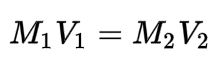Request a Tool

Dilution Factor Calculator

Dilution is the process of decreasing the concentration of a solution

Output

Total to stock
0
Dilutant to stock
0

Formula• V1 = Volume of solution before dilution
• V2 = Volume of solution after dilution
• M1 = Concentration before dilution
• M2 = Concentration after dilution

Defination / Uses

The dilution factor is the notation used to express how much of the original stock solution is present in the total solution, after dilution.

It will only be displayed as a ratio in this calculator. The parts of the stock solution to the parts of the dilutant added (S:D) or the parts of the stock solution to the parts of the total solution (S:T) are described in two ways, depending on whether the dilution factor is a ratio or an exponent (S:T).

Simple dilutions, in which a unit volume of a liquid substance is mixed with an appropriate volume of a solvent liquid to achieve the desired concentration, are commonly applied. Use the upper given formula for manual calculations. No sign-up, registration OR captcha is required to use this tool.